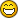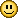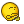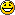# Maths teaser## Recommended PostsDO NOT RUIN THIS IF YOU HAVE STUDIED MATHS

Theorem: 3=4

Proof:

Assume:

a + b = c

This can also be written as:

4a - 3a + 4b - 3b = 4c - 3c

After reorganising:

4a + 4b - 4c = 3a + 3b - 3c

Take the constants out of the brackets:

4 * (a+b-c) = 3 * (a+b-c)

Remove the same term left and right:

4 = 3

How is this possible?

##### Share on other sites12

##### Share on other sites

I know.  :winking:

##### Share on other sitesI don't see how anyone who HASN'T studied maths would want to do it, though!  tongue.gif

136

##### Share on other sites

DO NOT RUIN THIS IF YOU HAVE STUDIED MATHS

Theorem: 3=4

Proof:

Assume:

a + b = c

This can also be written as:

4a - 3a + 4b - 3b = 4c - 3c

After reorganising:

4a + 4b - 4c = 3a + 3b - 3c

Take the constants out of the brackets:

4 * (a+b-c) = 3 * (a+b-c)

Remove the same term left and right:

4 = 3

How is this possible?

a+b=c

Therefore a+b-c=0

Therefore you're dividing 4(a+b-c)=3(a+b-c) by 0

Which isn't really on.

Infinity = Infinity.

##### Share on other sitesDO NOT RUIN THIS IF YOU HAVE STUDIED MATHS

Theorem: 3=4

Proof:

Assume:

a + b = c

This can also be written as:

4a - 3a + 4b - 3b = 4c - 3c

After reorganising:

4a + 4b - 4c = 3a + 3b - 3c

Take the constants out of the brackets:

4 * (a+b-c) = 3 * (a+b-c)

Remove the same term left and right:

4 = 3

How is this possible?

a+b=c

Therefore a+b-c=0

Therefore you're dividing 4(a+b-c)=3(a+b-c) by 0

Which isn't really on.

Infinity = Infinity.

http://www.francesandcompany.com/asicrystal/Star1/S432_Electroplated_Gold_Star_WoodenBase.jpg##### Share on other sitesNext one,

Does a proof exist that shows that 0.9999999999(recurring)=1

?

##### Share on other sites

Next one,

Does a proof exist that shows that 0.9999999999(recurring)=1

?

If it does, its a load of shite. You can't use Algebra to hide from infinity! rtfm.gif

##### Share on other siteshaha, i've already finished my six assignments for this week. Mainly on the poisson process, maximum likelihood estimates, graph theory, chaos theory, Transformations of random variables, the Cramér-Rao inequality etc.....##### Share on other sites

Next one,

Does a proof exist that shows that 0.9999999999(recurring)=1

?

Yes.  bluebiggrin.gif

Based on the fact it would be the shittest maths 'teaser' in history if the answer was 'no'.

##### Share on other sitesx=0.99999999999999999999999999

10x=9.9999999999999999999999999999

therefore 10x - x = 9

9x=9

x=1

##### Share on other sites

x=0.99999999999999999999999999

10x=9.9999999999999999999999999999

therefore 10x - x = 9

9x=9

x=1

blatant lies.

##### Share on other sites

10x=9.9999999999999999999999999999

I don't agree with this. You can't mess around with an infinite number of 9s like that nono.gif

You can't use Algebra to proove things that are blatantly false. 4 will never equal 3 just the same as .9.. will never equal 1.##### Share on other sites2+2=?

##### Share on other sitesExample

Convert 0.142857142857... into a fraction.

Let x = 0.142857142857...

We want to move the decimal point to the right, so that the first "block" of repeated digits appears before the decimal point. Remember that multiplying by 10 moves the decimal point 1 position to the right.

So in this example, we need to move the decimal point 6 places to the right (so we multiply both sides by 1 000 000):

1000000x = 142857.142857142857...

Now we can subtract our original number, x, from both sides to get rid of everything after the decimal point on the right:

1000000x - x = 142857

So 999999x = 142857

x = 142857/999999

= 1/7

##### Share on other sites

Example

Convert 0.142857142857... into a fraction.

Let x = 0.142857142857...

We want to move the decimal point to the right, so that the first "block" of repeated digits appears before the decimal point. Remember that multiplying by 10 moves the decimal point 1 position to the right.

So in this example, we need to move the decimal point 6 places to the right (so we multiply both sides by 1 000 000):

1000000x = 142857.142857142857...

Now we can subtract our original number, x, from both sides to get rid of everything after the decimal point on the right:

1000000x - x = 142857

So 999999x = 142857

x = 142857/999999

= 1/7

That bird of yours has gone to bed hasn't she?

##### Share on other sitesExample

Convert 0.142857142857... into a fraction.

Let x = 0.142857142857...

We want to move the decimal point to the right, so that the first "block" of repeated digits appears before the decimal point. Remember that multiplying by 10 moves the decimal point 1 position to the right.

So in this example, we need to move the decimal point 6 places to the right (so we multiply both sides by 1 000 000):

1000000x = 142857.142857142857...

Now we can subtract our original number, x, from both sides to get rid of everything after the decimal point on the right:

1000000x - x = 142857

So 999999x = 142857

x = 142857/999999

= 1/7

That bird of yours has gone to bed hasn't she?

She's in Middlesbrough planning our next integral of 2xdx between the limits of 13 and 10.

(That's our next 69 for those of you who can't evaluate that)

##### Share on other sites

How long have you been waiting to post that?##### Share on other sites'no, no, you need to move around around 6 degrees to the left and then adjust your hips upwards by (y+z)x centimetres!'

##### Share on other sites

For when people are done with whatever one you're on...

I was doing a maths paper this week (GCSE practise) and ended up with this bastard of a question that i couldn't make head nor tails out of.

5a + 3b = 9

2a - 3b = 12

What is a?

What is b?

I kinda worked out that 'b' must be a minece but got nowhere. Anyhow, resulted in me losing the three marks and a rollocking from the teacher. Stupid fat bitch didn't tell me how i should have done it, just pulled the paper away from me and told me to sit down. Great!

So, when you're done people, if you will ^^^ . :wink:

##### Share on other sites

But the number .9999.... doesn't really exist. An infinite number of 9s? Infinity does not exist in any real sense of the word, its just a philosophical concept. I don't see how you can just pick up a dot and drop it one place further along a line when the distance between this said dot in relation to the right hand edge of this line (The one way over there ~~~~~~~~~~~~~~~~~~~>) is immeasurable. Its too simplistic.

Maths is a load of shite.

##### Share on other sitesBut the number .9999.... doesn't really exist. An infinite number of 9s? Infinity does not exist in any real sense of the word, its just a philosophical concept. I don't see how you can just pick up a dot and drop it one place further along a line when the distance between this said dot in relation to the right hand edge of this line (The one way over there ~~~~~~~~~~~~~~~~~~~>) is immeasurable. Its too simplistic.

Maths is a load of shite.

Prove it.

##### Share on other sites

For when people are done with whatever one you're on...

I was doing a maths paper this week (GCSE practise) and ended up with this bastard of a question that i couldn't make head nor tails out of.

5a + 3b = 9

2a - 3b = 12

What is a?

What is b?

I kinda worked out that 'b' must be a minece but got nowhere. Anyhow, resulted in me losing the three marks and a rollocking from the teacher. Stupid fat bitch didn't tell me how i should have done it, just pulled the paper away from me and told me to sit down. Great!

So, when you're done people, if you will ^^^ . :wink:

a=3

b=-2

:roll:

PS. Minece?? Fucking hell mate